# LSGNT

## StaffProf Alessio Corti

Director and Mental Health Champion
a [dot] corti [at] imperial [dot] ac [dot] uk
Research interests
Geometry (high-dimensional birational geometry).Prof Michael Singer

Principal Investigator
michael [dot] singer [at] ucl [dot] ac [dot] uk
Research interests
Differential GeometryDr Lars Louder

Co-Director First Year
l [dot] louder [at] ucl [dot] ac [dot] uk
Research interests
Geometric group theory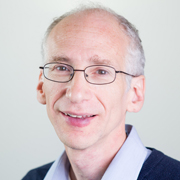Prof Fred Diamond

Co-Director for Years 2-4
fred [dot] diamond [at] kcl [dot] ac [dot] uk
Research interests
Modular forms, Galois representations, mod p Langlands, generalizations of Serre's conjecture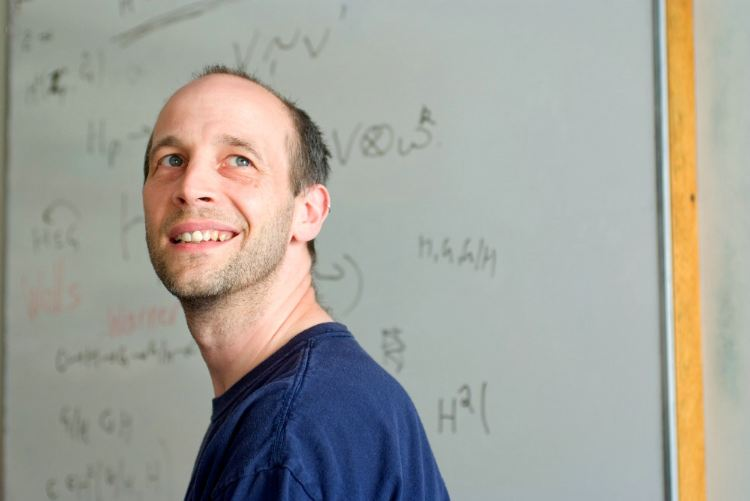Prof Kevin Buzzard

Co-Director Publicity and Recruitment
k [dot] buzzard [at] imperial [dot] ac [dot] uk
Research interests
Formal proof verification in number theory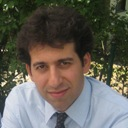Prof Payman Kassaei

Management Committee Member
payman [dot] l_kassaei [at] kcl [dot] ac [dot] uk
Research interests
Arithmetic geometry, (p-adic) automorphic forms, Shimura varieties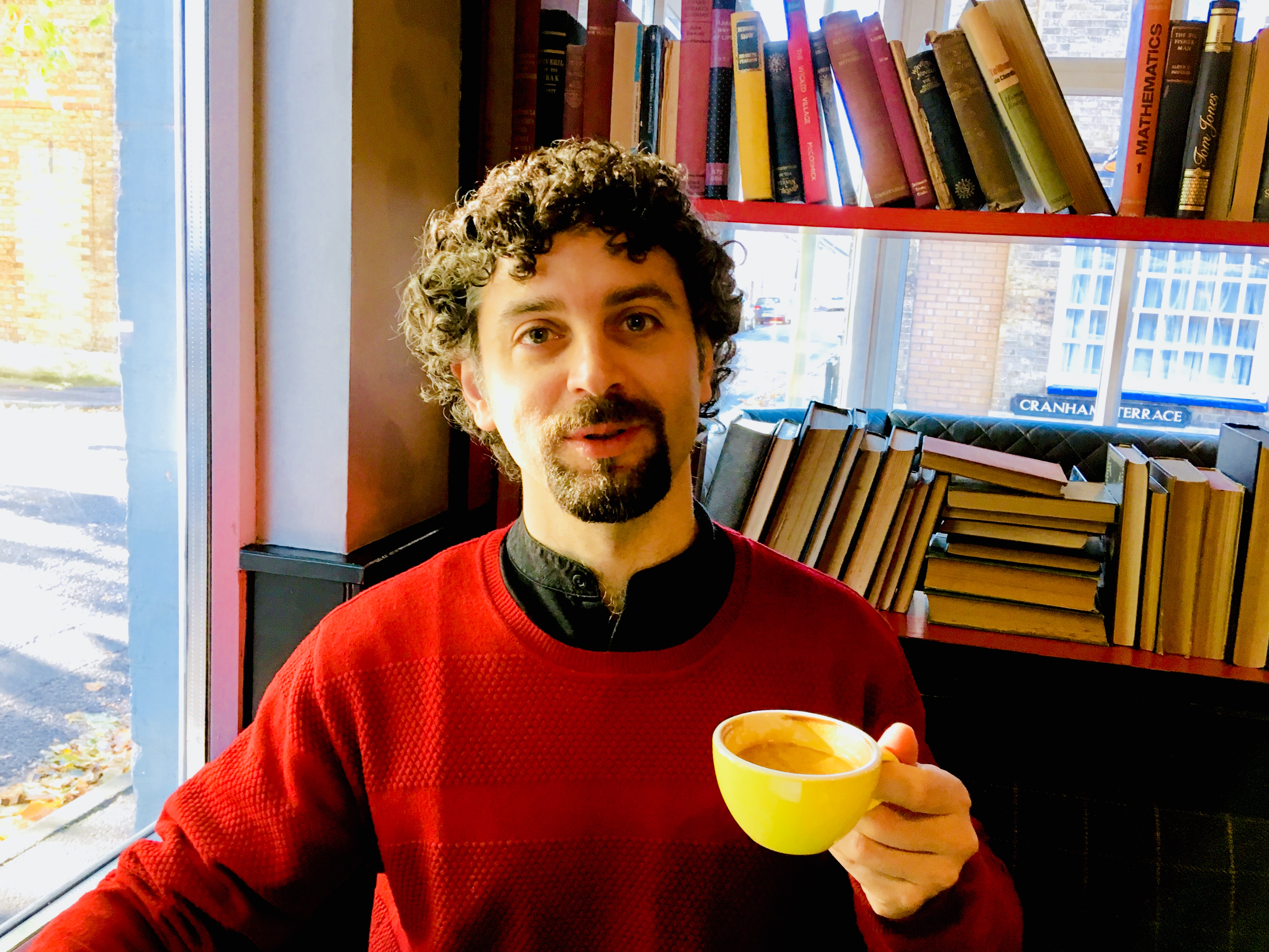Dr Costante Bellettini

Management Committee member
c [dot] bellettini [at] ucl [dot] ac [dot] uk
Research interests
Geometric measure theory, Geometric analysis, Calculus of variations and Elliptic PDEs.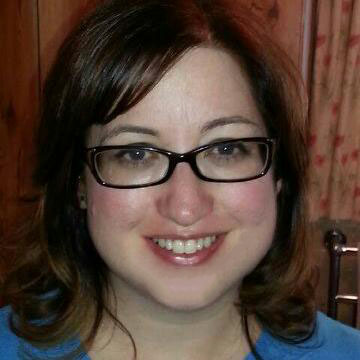Nicky Townsend

Centre Manager
enquiries [dot] lsgnt [at] ucl [dot] ac [dot] uk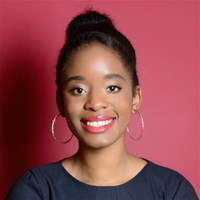Ms Kay Musonda

k [dot] musonda [at] ucl [dot] ac [dot] uk

Name Institution Email Subject Research interests
Prof Michael Singer University College London michael [dot] singer [at] ucl [dot] ac [dot] uk Geometry Differential Geometry
Prof Alexei Skorobogatov Imperial College London a [dot] skorobogatov [at] imperial [dot] ac [dot] uk Geometry Arithmetic Geometry, Rational Points
Prof Jurgen Berndt King's College London jurgen [dot] berndt [at] kcl [dot] ac [dot] uk Geometry Special structures, exceptional geometries, symmetric and homogeneous spaces
Prof Simon Donaldson Imperial College London s [dot] donaldson [at] imperial [dot] ac [dot] uk Geometry Differential and algebraic geometry; global analysis
Dr Jonny Evans University College London j [dot] d [dot] evans [at] ucl [dot] ac [dot] uk Geometry Symplectic Topology
Prof Rod Halburd University College London r [dot] halburd [at] ucl [dot] ac [dot] uk Geometry Integrable systems, singularities of differential equations
Dr Gustav Holzegel Imperial College London g [dot] holzegel [at] imperial [dot] ac [dot] uk Geometry Differential geometry and geometric analysis; general relativity
Prof Simon Salamon King's College London simon [dot] salamon [at] kcl [dot] ac [dot] uk Geometry Differential geometry
Dr Felix Schulze University College London f [dot] schulze [at] ucl [dot] ac [dot] uk Geometry Geometric analysis
Prof Simon Scott King's College London simon [dot] scott [at] kcl [dot] ac [dot] uk Geometry Topology, analysis
Prof Nick Shepherd-Barron King's College London nicholas [dot] shepherd-barron [at] kcl [dot] ac [dot] uk Geometry Algebraic geometry
Prof Richard Thomas Imperial College London richard [dot] thomas [at] imperial [dot] ac [dot] uk Geometry Algebraic geometry (mirror symmetry, moduli problems)
Dr Travis Schedler Imperial College London t [dot] schedler [at] imperial [dot] ac [dot] uk Geometry Algebra, Noncommutative and Poisson Geometry, Representation Theory
Prof Dmitri Vassiliev University College London d [dot] vassiliev [at] ucl [dot] ac [dot] uk Geometry Spectral theory, microlocal analysis, geometric analysis and general relativity
Dr Mahir Hadžić University College London m [dot] hadzic [at] ucl [dot] ac [dot] uk Geometry Nonlinear partial differential equations and general relativity, free boundary problems and singularity formation phenomena.
Dr John Armstrong King's College London john [dot] 1 [dot] armstrong [at] kcl [dot] ac [dot] uk Geometry Financial mathematics, Geometry and finance and Information geometry.
Dr Davoud Cheraghi Imperial College London d [dot] cheraghi [at] imperial [dot] ac [dot] uk Geometry Complex Geometry (invariant complex structures, moduli spaces of rational functions).
Dr Ben Lambert (post doc) University College London b [dot] lambert [at] ucl [dot] ac [dot] uk Geometry
Prof Xue-Mei Li Imperial College London mei [dot] li [at] imperial [dot] ac [dot] uk Geometry Parabolic PDEs, Hoermander type operators, Geometry of Stochastic processes, Stochastic perturbation and dynamical systems, Analysis on path spaces.
Dr Ed Segal University College London e [dot] segal [at] ucl [dot] ac [dot] uk Geometry Algebraic geometry and homological algebra
Prof Tom Coates Imperial College London t [dot] coates [at] imperial [dot] ac [dot] uk Geometry Algebraic Geometry (Gromov-Witten Theory, Mirror Symmetry)
Prof Konstanze Rietsch King's College London konstanze [dot] rietsch [at] kcl [dot] ac [dot] uk Geometry Lie Theory and Mirror Symmetry
Dr Marie-Amelie Lawn (Strategic Teaching Fellow) Imperial College London m [dot] lawn [at] imperial [dot] ac [dot] uk Geometry Lorentzian and Pseudo-Riemannian Geometry, Submanifold Theory, Spin Geometry
Prof Jason Lotay (Honorary Senior Research Associate) University College London j [dot] lotay [at] ucl [dot] ac [dot] uk Geometry Differential geometry and geometric analysis. Professor Lotay will move to the University of Oxford in January 2019, but will remain in an honorary position at UCL.
Dr Lorenzo Foscolo University College London l [dot] foscolo [at] ucl [dot] ac [dot] uk Geometry Differential Geometry
Dr Martin Taylor Imperial College London martin [dot] taylor [at] imperial [dot] ac [dot] uk Geometry General relativity, geometric analysis, nonlinear partial differential equations.
Asso Jeffrey Galkowski University College London j [dot] galkowski [at] ucl [dot] ac [dot] uk Geometry Spectral geometry, scattering theory, microlocal analysis and applications of dynamical systems
Dr John Talbot University College London j [dot] talbot [at] ucl [dot] ac [dot] uk Geometry Combinatorics, extremal problems for hypergraphs and graphs
Dr Alexey Pokrovskiy University College London a [dot] pokrovskiy [at] ucl [dot] ac [dot] uk Geometry Combinatorics, Extremal Graph Theory and Latin Squares
Prof Paolo Cascini Imperial College London p [dot] cascini [at] imperial [dot] ac [dot] uk Geometry Algebraic geometry (birational geometry, minimal model program)
Prof Giuseppe Tinaglia King's College London giuseppe [dot] tinaglia [at] kcl [dot] ac [dot] uk Geometry Differential Geometry
Dr Yanki Lekili Imperial College London y [dot] lekili [at] imperial [dot] ac [dot] uk Geometry Symplectic topology, mirror symmetry
Prof Dmitri Panov King's College London dmitri [dot] panov [at] kcl [dot] ac [dot] uk Geometry Symplectic, complex and hyperbolic geometry
Dr Anthea Monod Imperial College London a [dot] monod [at] imperial [dot] ac [dot] uk Geometry Applied and computational algebraic topology; applied and computational algebraic geometry; metric geometry; statistics and machine learning; mathematical biology
Dr Mehdi Yazdi King's College London mehdi [dot] yazdi [at] kcl [dot] ac [dot] uk Geometry Low-dimensional topology and geometry, foliations, and mapping class groups of surfaces.
Dr Calum Spicer King's College London calum [dot] spicer [at] kcl [dot] ac [dot] uk Geometry Algebraic geometry, with a particular focus on birational geometry and holomorphic foliations.
Dr Steven Sivek (UCL Honorary Associate Professor) Imperial College London s [dot] sivek [at] imperial [dot] ac [dot] uk Geometry Low-dimensional topology, symplectic geometry, and gauge theory.
Dr Nikon Kurnosov University College London n [dot] kurnosov [at] ucl [dot] ac [dot] uk Geometry Hyperkahler geometry, Algebraic geometry
Prof David Burns King's College London david [dot] burns [at] kcl [dot] ac [dot] uk Number Theory Iwasawa theory, Tamagawa number conjectures, Stark's conjecture and refinements
Dr David Helm Imperial College London d [dot] helm [at] imperial [dot] ac [dot] uk Number Theory Number theory and representation theory of p-adic groups
Dr Richard Hill University College London ucahrml [at] ucl [dot] ac [dot] uk Number Theory Cohomology of arithmetic groups
Dr Ambrus Pal Imperial College London a [dot] pal [at] imperial [dot] ac [dot] uk Number Theory Arithmetic algebraic geometry
Prof Andrei Yafaev University College London a [dot] yafaev [at] ucl [dot] ac [dot] uk Number Theory Arithmetic algebraic geometry
Dr Ana Caraiani Imperial College London a [dot] caraiani [at] imperial [dot] ac [dot] uk Number Theory My interests include the classical and p-adic Langlands programs, Shimura varieties, and arithmetic geometry.
Dr Lucio Guerberoff (Post doc) University College London l [dot] guerberoff [at] ucl [dot] ac [dot] uk Number Theory Number theory, automorphic forms and Galois representations.
Prof Toby Gee Imperial College London toby [dot] gee [at] imperial [dot] ac [dot] uk Number Theory Galois representations and automorphic forms
Dr Owen Patashnick (Senior Research Fellow) King's College London owen [dot] patashnick [at] kcl [dot] ac [dot] uk Number Theory Arithmetic Geometry; periods, motives, algebraic cycles and rational homotopy theory.
Prof Sarah Zerbes University College London s [dot] zerbes [at] ucl [dot] ac [dot] uk Number Theory p-adic Hodge theory and Iwasawa theory
Dr Cecilia Busuioc University College London cecilia [dot] busuioc [at] ucl [dot] ac [dot] uk Number Theory L functions
Prof Vladimir Dokchitser University College London v [dot] dokchitser [at] ucl [dot] ac [dot] uk Number Theory Abelian varieties, L-values, Galois representations
Dr Ian Petrow University College London i [dot] petrow [at] ucl [dot] ac [dot] uk Number Theory Analytic number theory, particularly the analytic theory of automorphic forms and L-functions.
Dr Luis Garcia Martinez University College London l [dot] e [dot] garcia [at] ucl [dot] ac [dot] uk Number Theory Automorphic forms, Shimura varieties and special values of L-functions.
Dr Steve Lester King's College London steve [dot] lester [at] kcl [dot] ac [dot] uk Number Theory Analytic number theory, L-functions, and automorphic forms.
Dr Netan Dogra King's College London netan [dot] dogra [at] kcl [dot] ac [dot] uk Number Theory Diophantine geometry and arithmetic fundamental groups
Prof Yiannis Petridis University College London i [dot] petridis [at] ucl [dot] ac [dot] uk Number Theory Analytic number theory, automorphic forms and spectral theory
Dr Ben Krause King's College London ben [dot] krause [at] kcl [dot] ac [dot] uk Number Theory Pointwise ergodic theory, harmonic analysis, additive combinatorics
Prof Sameer Murthy King's College London sameer [dot] murthy [at] kcl [dot] ac [dot] uk Number Theory String theory and black holes. Applications of modular forms and automorphic forms in physics.
Prof Igor Wigman King's College London igor [dot] wigman [at] kcl [dot] ac [dot] uk Number Theory Analytic number theory, probability and stochastic processes, quantum chaos, spectral geometry.
Dr Rachel Newton King's College London rachel [dot] newton [at] kcl [dot] ac [dot] uk Number Theory Rational points on algebraic varieties
Dr Johannes Nicaise Imperial College London j [dot] nicaise [at] imperial [dot] ac [dot] uk Both Arithmetic, Algebraic and Non-Archimedean Geometry